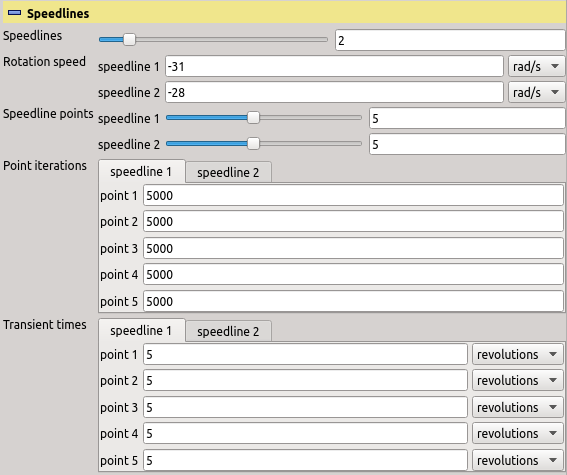Previous: Components Up: TCFD – GUI Setup Next: Turbulence
This is an automatically generated documentation by LaTeX2HTML utility. In case of any issue, please, contact us at info@cfdsupport.com.

### Speedlines

Every simulation consists of individual points with similar setup, which are grouped into speedlines sharing the rotation speed. Number of speedlines and number of points in every speedline is chosen in this section. Some other sections then adjust number of their inputs according to the numbers chosen here. The particular value of the rotation speed is set in “Reference frames” section.

• The slider “Speedlines” sets the number of speedlines (groups of points with common rotation speed).

• The sliders in “Speedline points” set the number of points in every speedline.

• The entries in “Point iterations” set the number of iterations of steady-state calculation used for each of the points. This actually sets the maximal number of iterations. If “Convergence check” is enabled, the solver may terminate even before the iteration count reaches this number, if it decides that the efficiency and the fluxes are sufficiently converged.

• The entries in “Transient times” set the time span of transient calculation used for each of the points. As in stationary calculation, if “Convergence check” is enabled, the solver may terminate even before the end of the requested time interval, if it decides that the efficiency and the fluxes are sufficiently converged.Figure: Plugin TCFDSource – speedline settings.